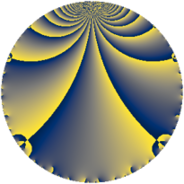# Properties

 Label 7056.2.a.pLevel $7056$ Weight $2$ Character orbit 7056.a Self dual yes Analytic conductor $56.342$ Analytic rank $0$ Dimension $1$ CM no Inner twists $1$

# Related objects

## Newspace parameters

 Level: $$N$$ $$=$$ $$7056 = 2^{4} \cdot 3^{2} \cdot 7^{2}$$ Weight: $$k$$ $$=$$ $$2$$ Character orbit: $$[\chi]$$ $$=$$ 7056.a (trivial)

## Newform invariants

 Self dual: yes Analytic conductor: $$56.3424436662$$ Analytic rank: $$0$$ Dimension: $$1$$ Coefficient field: $$\mathbb{Q}$$ Coefficient ring: $$\mathbb{Z}$$ Coefficient ring index: $$1$$ Twist minimal: no (minimal twist has level 21) Fricke sign: $$-1$$ Sato-Tate group: $\mathrm{SU}(2)$

## $q$-expansion

 $$f(q)$$ $$=$$ $$q - 2q^{5} + O(q^{10})$$ $$q - 2q^{5} + 4q^{11} + 2q^{13} - 6q^{17} + 4q^{19} - q^{25} + 2q^{29} + 6q^{37} + 2q^{41} + 4q^{43} - 6q^{53} - 8q^{55} - 12q^{59} + 2q^{61} - 4q^{65} - 4q^{67} + 6q^{73} + 16q^{79} + 12q^{83} + 12q^{85} - 14q^{89} - 8q^{95} - 18q^{97} + O(q^{100})$$

## Embeddings

For each embedding $$\iota_m$$ of the coefficient field, the values $$\iota_m(a_n)$$ are shown below.

For more information on an embedded modular form you can click on its label.

Label $$\iota_m(\nu)$$ $$a_{2}$$ $$a_{3}$$ $$a_{4}$$ $$a_{5}$$ $$a_{6}$$ $$a_{7}$$ $$a_{8}$$ $$a_{9}$$ $$a_{10}$$
1.1
 0
0 0 0 −2.00000 0 0 0 0 0
 $$n$$: e.g. 2-40 or 990-1000 Significant digits: Format: Complex embeddings Normalized embeddings Satake parameters Satake angles

## Atkin-Lehner signs

$$p$$ Sign
$$2$$ $$-1$$
$$3$$ $$-1$$
$$7$$ $$-1$$

## Inner twists

This newform does not admit any (nontrivial) inner twists.

## Twists

By twisting character orbit
Char Parity Ord Mult Type Twist Min Dim
1.a even 1 1 trivial 7056.2.a.p 1
3.b odd 2 1 2352.2.a.v 1
4.b odd 2 1 441.2.a.f 1
7.b odd 2 1 1008.2.a.l 1
12.b even 2 1 147.2.a.a 1
21.c even 2 1 336.2.a.a 1
21.g even 6 2 2352.2.q.x 2
21.h odd 6 2 2352.2.q.e 2
24.f even 2 1 9408.2.a.bv 1
24.h odd 2 1 9408.2.a.m 1
28.d even 2 1 63.2.a.a 1
28.f even 6 2 441.2.e.a 2
28.g odd 6 2 441.2.e.b 2
56.e even 2 1 4032.2.a.h 1
56.h odd 2 1 4032.2.a.k 1
60.h even 2 1 3675.2.a.n 1
84.h odd 2 1 21.2.a.a 1
84.j odd 6 2 147.2.e.b 2
84.n even 6 2 147.2.e.c 2
105.g even 2 1 8400.2.a.bn 1
140.c even 2 1 1575.2.a.c 1
140.j odd 4 2 1575.2.d.a 2
168.e odd 2 1 1344.2.a.g 1
168.i even 2 1 1344.2.a.s 1
252.s odd 6 2 567.2.f.g 2
252.bi even 6 2 567.2.f.b 2
308.g odd 2 1 7623.2.a.g 1
336.v odd 4 2 5376.2.c.r 2
336.y even 4 2 5376.2.c.l 2
420.o odd 2 1 525.2.a.d 1
420.w even 4 2 525.2.d.a 2
924.n even 2 1 2541.2.a.j 1
1092.d odd 2 1 3549.2.a.c 1
1428.b odd 2 1 6069.2.a.b 1
1596.p even 2 1 7581.2.a.d 1

By twisted newform orbit
Twist Min Dim Char Parity Ord Mult Type
21.2.a.a 1 84.h odd 2 1
63.2.a.a 1 28.d even 2 1
147.2.a.a 1 12.b even 2 1
147.2.e.b 2 84.j odd 6 2
147.2.e.c 2 84.n even 6 2
336.2.a.a 1 21.c even 2 1
441.2.a.f 1 4.b odd 2 1
441.2.e.a 2 28.f even 6 2
441.2.e.b 2 28.g odd 6 2
525.2.a.d 1 420.o odd 2 1
525.2.d.a 2 420.w even 4 2
567.2.f.b 2 252.bi even 6 2
567.2.f.g 2 252.s odd 6 2
1008.2.a.l 1 7.b odd 2 1
1344.2.a.g 1 168.e odd 2 1
1344.2.a.s 1 168.i even 2 1
1575.2.a.c 1 140.c even 2 1
1575.2.d.a 2 140.j odd 4 2
2352.2.a.v 1 3.b odd 2 1
2352.2.q.e 2 21.h odd 6 2
2352.2.q.x 2 21.g even 6 2
2541.2.a.j 1 924.n even 2 1
3549.2.a.c 1 1092.d odd 2 1
3675.2.a.n 1 60.h even 2 1
4032.2.a.h 1 56.e even 2 1
4032.2.a.k 1 56.h odd 2 1
5376.2.c.l 2 336.y even 4 2
5376.2.c.r 2 336.v odd 4 2
6069.2.a.b 1 1428.b odd 2 1
7056.2.a.p 1 1.a even 1 1 trivial
7581.2.a.d 1 1596.p even 2 1
7623.2.a.g 1 308.g odd 2 1
8400.2.a.bn 1 105.g even 2 1
9408.2.a.m 1 24.h odd 2 1
9408.2.a.bv 1 24.f even 2 1

## Hecke kernels

This newform subspace can be constructed as the intersection of the kernels of the following linear operators acting on $$S_{2}^{\mathrm{new}}(\Gamma_0(7056))$$:

 $$T_{5} + 2$$ $$T_{11} - 4$$ $$T_{13} - 2$$ $$T_{17} + 6$$ $$T_{23}$$

## Hecke characteristic polynomials

$p$ $F_p(T)$
$2$ $$T$$
$3$ $$T$$
$5$ $$2 + T$$
$7$ $$T$$
$11$ $$-4 + T$$
$13$ $$-2 + T$$
$17$ $$6 + T$$
$19$ $$-4 + T$$
$23$ $$T$$
$29$ $$-2 + T$$
$31$ $$T$$
$37$ $$-6 + T$$
$41$ $$-2 + T$$
$43$ $$-4 + T$$
$47$ $$T$$
$53$ $$6 + T$$
$59$ $$12 + T$$
$61$ $$-2 + T$$
$67$ $$4 + T$$
$71$ $$T$$
$73$ $$-6 + T$$
$79$ $$-16 + T$$
$83$ $$-12 + T$$
$89$ $$14 + T$$
$97$ $$18 + T$$